# Chapter # 10 : Spanning Tree & Types | Kruskal’s Algorithm | Prim’s Algorithm | Dijkstra’s Algorithm

## Minimum Spanning Tree

What is a Spanning Tree?

A spanning tree is a subset of Graph G, which has all the vertices covered with a minimum possible number of edges. The spans G (that is, it includes every vertex of G) and is a subgraph of G (every edge in the tree belongs to G)

By this definition, we can draw a conclusion that every connected and undirected Graph G has at least one spanning tree. A disconnected graph does not have any spanning tree, as it cannot be spanned to all its vertices.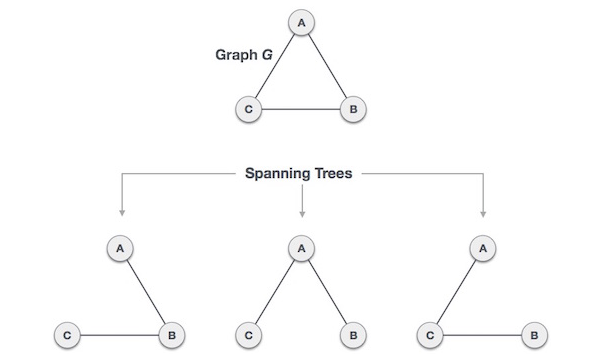Figure 1​​ Converting Graph into spanning tree

Properties of Spanning Tree?

• A connected graph G can have more than one spanning tree.

• All possible spanning trees of graph G, have the same number of edges and vertices.

• The spanning tree does not have any cycle (loops).

• Removing one edge from the spanning tree will make the graph disconnected, i.e. the spanning tree is minimally connected.

• Adding one edge to the spanning tree will create a circuit or loop, i.e. the spanning tree is maximally acyclic.

Minimum Spanning Tree (MST)

In a weighted graph, a minimum spanning tree is a spanning tree that has minimum weight than all other spanning trees of the same graph. In real-world situations, this weight can be measured as distance, congestion, traffic load or any arbitrary value denoted to the edges.

Most important spanning tree algorithms are:

• Kruskal's Algorithm

• Prim’s Algorithm

Kruskal's Algorithm:

Kruskal's algorithm to find the minimum cost spanning tree uses the greedy approach. This algorithm treats the graph as a forest and every node it has as an individual tree. A tree connects to another only and only if, it has the least cost among all available options and does not violate MST properties

To understand Kruskal's algorithm let us consider the following example.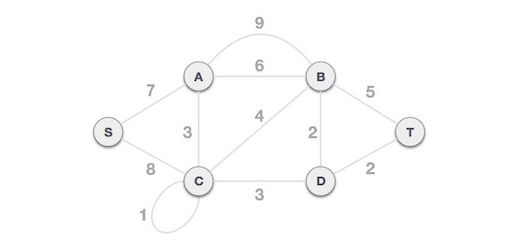## Step 1 - Remove all loops and Parallel Edges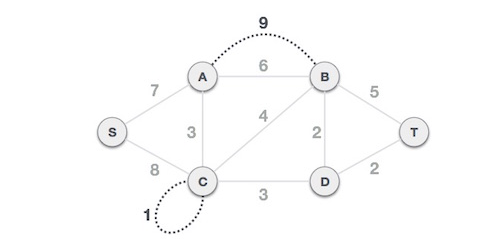In case of parallel edges, keep the one which has the least cost associated and remove all others.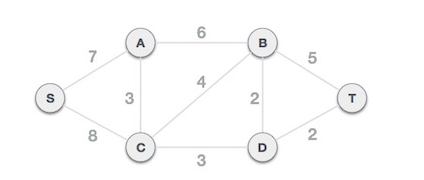## Step 2 - Arrange all edges in their increasing order of weight

The next step is to create a set of edges and weight, and arrange them in an ascending order of weightage (cost).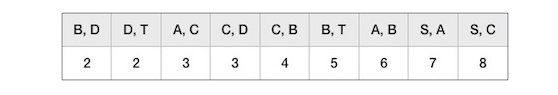## ​​ Step 3 - Add the edge which has the least weightage

Now we start adding edges to the graph beginning from the one which has the least weight. Throughout, we shall keep checking that the spanning properties remain intact. In case, by adding one edge, the spanning tree property does not hold then we shall consider not to include the edge in the graph.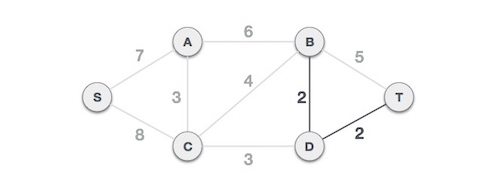The least cost is 2 and edges involved are B,D and D,T. We add them. Adding them does not violate spanning tree properties, so we continue to our next edge selection.

Next cost is 3, and associated edges are A,C and C,D. We add them again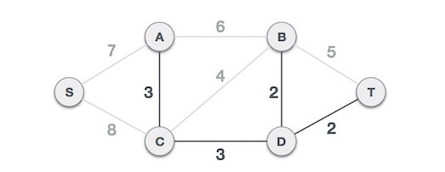Next cost in the table is 4, and we observe that adding it will create a circuit in the graph.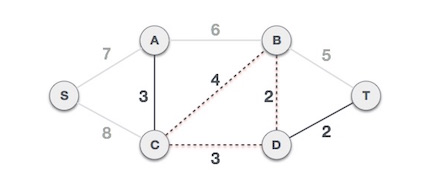We ignore it. In the process we shall ignore/avoid all edges that create a circuit.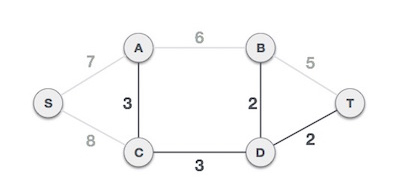We observe that edges with cost 5 and 6 also create circuits. We ignore them and move on.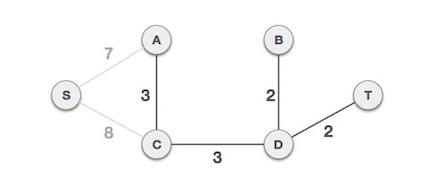Now we are left with only one node to be added. Between the two least cost edges available 7 and 8, we shall add the edge with cost 7.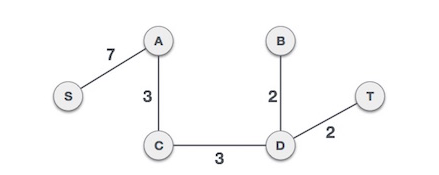By adding edge S, A we have included all the nodes of the graph and we now have minimum cost spanning tree.

Prim’s Algorithm:

Prim's algorithm to find minimum cost spanning tree (as Kruskal's algorithm) uses the greedy approach. Prim's algorithm shares a similarity with the shortest path first algorithms.

Prim's algorithm, in contrast with Kruskal's algorithm, treats the nodes as a single tree and keeps on adding new nodes to the spanning tree from the given graph.

To contrast with Kruskal's algorithm and to understand Prim's algorithm better, we shall use the same example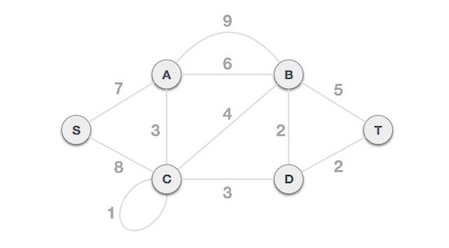## Step 1 - Remove all loops and parallel edges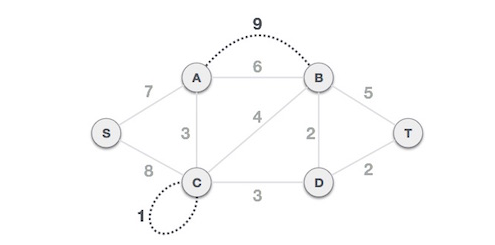Remove all loops and parallel edges from the given graph. In case of parallel edges, keep the one which has the least cost associated and remove all others.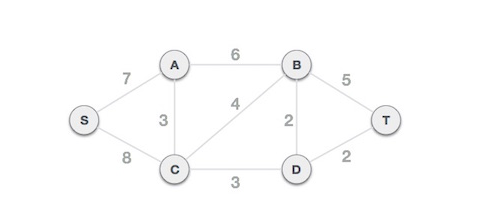## Step 2 - Choose any arbitrary node as root node

In this case, we choose S node as the root node of Prim's spanning tree. This node is arbitrarily chosen, so any node can be the root node. One may wonder why any video can be a root node. So the answer is, in the spanning tree all the nodes of a graph are included and because it is connected then there must be at least one edge, which will join it to the rest of the tree.

## Step 3 - Check outgoing edges and select the one with less cost

After choosing the root node S, we see that S,A and S,C are two edges with weight 7 and 8, respectively. We choose the edge S,A as it is lesser than the other.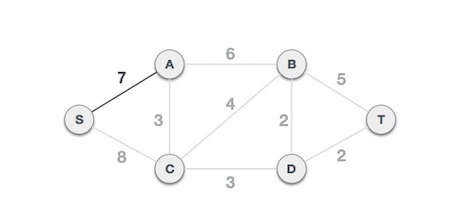Now, the tree S-7-A is treated as one node and we check for all edges going out from it. We select the one which has the lowest cost and include it in the tree.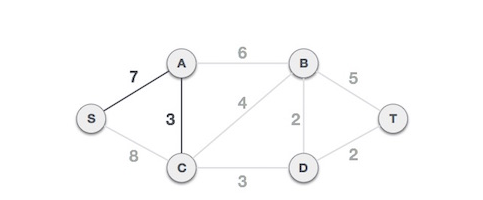After this step, S-7-A-3-C tree is formed. Now we'll again treat it as a node and will check all the edges again. However, we will choose only the least cost edge. In this case, C-3-D is the new edge, which is less than other edges' cost 8, 6, 4, etc.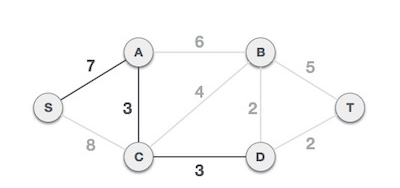After adding node D to the spanning tree, we now have two edges going out of it having the same cost, i.e. D-2-T and D-2-B. Thus, we can add either one. But the next step will again yield edge 2 as the least cost. Hence, we are showing a spanning tree with both edges included.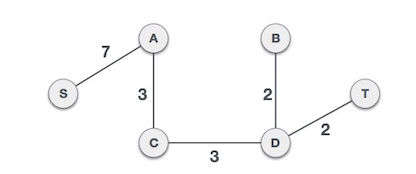We may find that the output spanning tree of the same graph using two different algorithms is same.

Dijkstra’s Algorithm:

The algorithm we are going to use to determine the shortest path is called “Dijkstra’s algorithm.” Dijkstra’s algorithm is an iterative algorithm that provides us with the shortest path from one particular starting node to all other nodes in the graph. Again this is similar to the results of a breadth first search.

Below are the detailed steps used in Dijkstra’s algorithm to find the shortest path from a single source vertex to all other vertices in the given graph.

• Algorithm
1) Create a set sptSet (shortest path tree set) that keeps track of vertices included in shortest path tree, i.e., whose minimum distance from source is calculated and finalized. Initially, this set is empty.
2) Assign a distance value to all vertices in the input graph. Initialize all distance values as INFINITE. Assign distance value as 0 for the source vertex so that it is picked first.
3) While sptSet doesn’t include all vertices
….a) Pick a vertex u which is not there in sptSetand has minimum distance value.
….b) Include u to sptSet.
….c) Update distance value of all adjacent vertices of u. To update the distance values, iterate through all adjacent vertices. For every adjacent vertex v, if sum of distance value of u (from source) and weight of edge u-v, is less than the distance value of v, then update the distance value of v.between the initial node and remaining unvisited nodes), then stop. The algorithm has finished.

• Otherwise, select the unvisited node that is marked with the smallest tentative distance, set it as the new "current node", and go back to step 3.

Let us understand with the following example: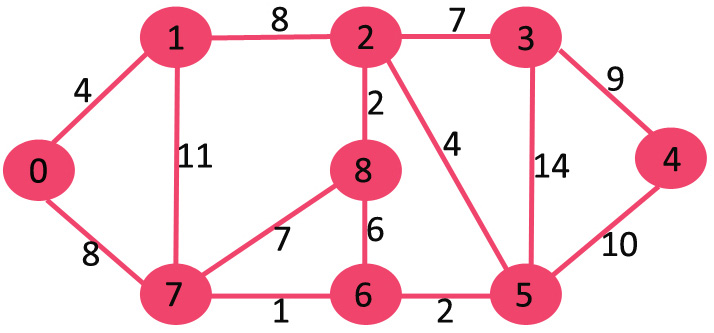Note* sptSet (shortest path tree set)

SPT (shortest path tree)

Considering 0 is starting node, pick the vertex with minimum distance value. Adjacent vertices of 0 are 1 and 7. The distance values of 1 and 7 are updated as 4 and 8. Following subgraph shows vertices and their distance values, only the vertices with finite distance values are shown. The vertices included in SPT are shown in green color.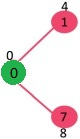Pick the vertex with minimum distance value and not already included in SPT (not in sptSET). The vertex 1 is picked and added to sptSet. So sptSet now becomes {0, 1}. Update the distance values of adjacent vertices of 1. The distance value of vertex 2 becomes 12.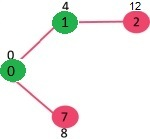Pick the vertex with minimum distance value and not already included in SPT (not in sptSET). Vertex 7 is picked. So sptSet now becomes {0, 1, 7}. Update the distance values of adjacent vertices of 7. The distance value of vertex 6 and 8 becomes finite (15 and 9 respectively)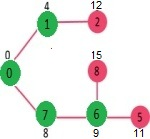We repeat the above steps until sptSet doesn’t include all vertices of given graph. Finally, we get the following Shortest Path Tree (SPT).